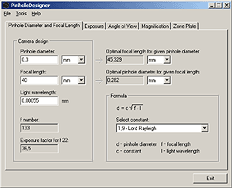Program window for calculating the optimal diameter of the pinhole and optimal focal length.Program window for calculating exposure times.

# PINHOLEDESIGNER 2.0

PinholeDesigner is a program for Windows and is aimed at making the calculations for designing and using pinhole cameras easier. Amongst its main features are calculations for the optimal diameter of the pinhole and the exposure times for pinhole cameras.

This program offers:

 – calculation of the optimal diameter of the pinhole – calculation of the optimal focal length – calculation of the f number for a given combination of pinhole and focal length – calculation of the exposure factor for f 22 – calculation of the exposure times for a given f number of the pinhole camera – calculation of the extended exposure times due to reciprocity failure for the majority of commonly used films – saving exposure table as Microsoft Excel file or text file – calculation of the angle of view, with diagram – calculation of the magnification and size of the subject on light-sensitive material – calculation of the zone plate with optional number of zones – calculation of the f number for the zone plate – saving the zone plate in Adobe PDF format – conversion of millimetres to inches and back